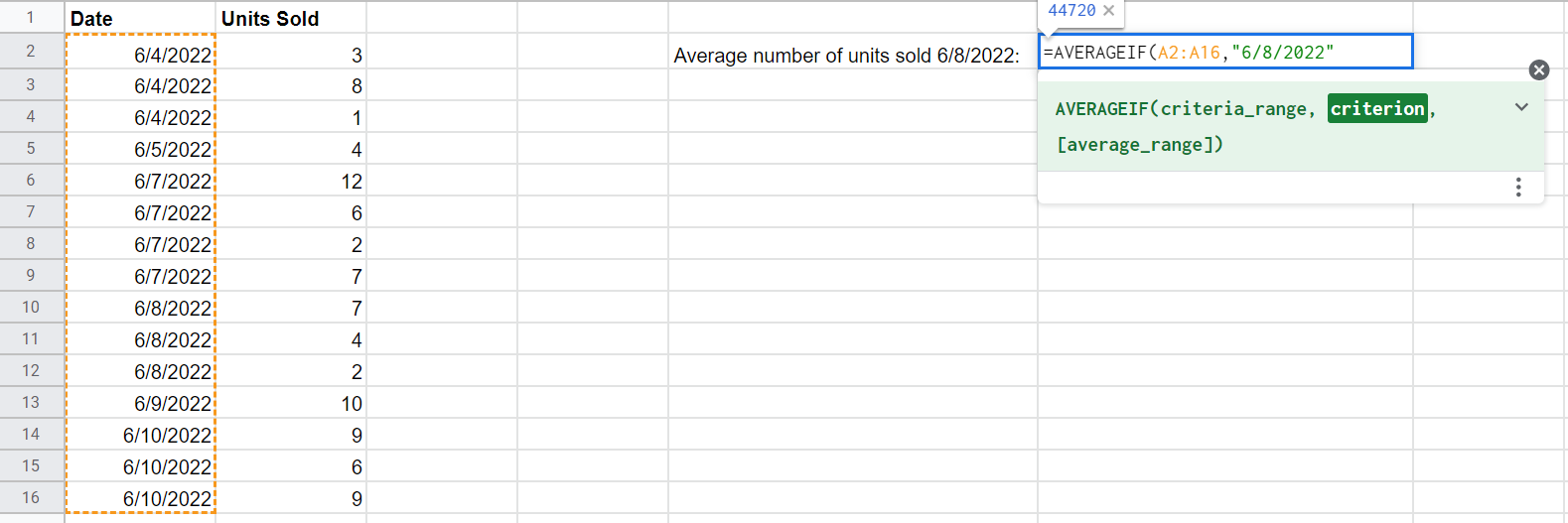# AVERAGEIF Google Sheets: The Ultimate Guide for 2023## The Google Sheets AVERAGE IF Function

The AVERAGEIF function in Google Sheets calculates the average of numbers within a range that meet a given set of criteria—combining the AVERAGE and IF functions.

### Syntax

=AVERAGEIF(criteria_range, criterion, [average_range])

Where:

criteria_range=

the data to which the criterion will be applied.

criterion=

the condition—a number, text, date, or comparison operator—in the criteria range you wish to specify.

[average_range]=

an optional argument, the average range indicates the numeric values you wish to average. If left blank, the average range defaults to the criteria range.

### AVERAGEIF function with text

When the criterion is set as text, the function is a great way to calculate the average of numbers associated with a particular word or group of words.

Let’s say you have a sheet identifying the average monthly sales for a set of businesses within a particular area. If you want to calculate the total average monthly sales for all the businesses in a given category—let’s say automotive—this function will come in handy.

#### Step 2: Select the range

With a small range, you can simply select the cells with your cursor. For a larger one, it will be easiest to just type it inside the function.

#### Step 3: Supply the criterion

When you’re using text as the criterion, you must place it in double quotation marks (“”) within the function. If you’re calculating the monthly sales for all automotive businesses, enter “Automotive.”

#### Step 4: Identify the average range

When your criterion is text, you’ll need to use the optional average range address reference to indicate the cells whose values you want to average.

### AVERAGEIF function with Numbers

The steps for using this function are a little different when the criterion is a number.

Let’s say a group of children between the ages of 8 and 10 were given a test, and you want to calculate the average score for the 8-year-olds.

#### Step 2: Select the range

In this instance, select B2 through B16, to identify the Age column as the range.

#### Step 3: Supply the criterion

When the criterion is a number value, simply enter the digit as the next argument—in this case, 8.

#### Step 4: Identify the average range

Here you would select C2 through C16 to average the relevant data in the test score column.

### AVERAGEIF function with Dates

The function can also be used with the criterion set as a date.

Say, for example, you want to use the formula to calculate the average number of units sold per customer on June 8, 2022.

#### Step 3: Supply the criterion

When you’re working with a date, enter the value in double quotation marks, as you would with text.Supplying a date as the criterion for Average If in sheet

### AVERAGEIF function with comparison operators

Finally, you can also calculate an average for a criterion determined by six comparison operators:

• Equals (=)
• Not equal to (<>)
• Greater than (>)
• Greater than or equal to (>=)
• Less than (<)
• Less than or equal to (<=)

Let’s say you’re comparing the nutritional info of different snacks and wish to determine the average grams of protein for the snacks with more than 100 calories.

#### Step 3: Supply the criterion

As with text and dates, criterion involving comparison operators must be entered surrounded by double quotation marks.

### FAQs

What if I have multiple multiple rangers or multiple criteria?

Google Sheets allows you to calculate the average for multiple ranges, criteria, and/or average ranges. To do so, however, you must use a different function with a distinct syntax: AVERAGEIFS.

### Related IF Functions

IF: Allows you to check for specific conditions across a dataset

SUMIF: Use to sum numbers if they meet a certain condition..

SUMIFS: Sums data from cells that meet multiple criteria

COUNTIF: count data if it fulfils certain criteria

COUNTIFS: Count data that fulfils two or more criteria.

COUNTIF Not Null: Count cells if they contain data

IFS: IFS is a more elegant way to evaluate data against multiple criteria.

IFERROR: Allows you to output values when an Error in a formula occurs

IF THEN: Allows you tor write statements that use IF X Then Y Logic

IF AND: Combines the functionality of the IF and AND functions

IF Else: Set conditions that give an output depending on whether a given condition is fulfilled or not

Multiple IF Statements: How to chain multiple statements together.

IF OR: Combines the functionality of the IF and OR functions

IF Contains: used to look for cells that contain a certain string as its value